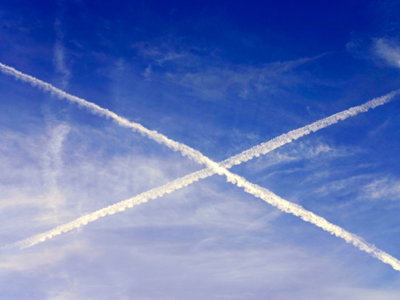Lines that cross each other are said to 'intersect'.

# Shapes 2 (Easy)

Geometry is a word we use in maths. It means the study of shapes. In this, the second of our Easy Eleven Plus maths quizzes on Shapes, we look at some of the terms we use in geometry, like vertex, polygon, radii and perimeter, for example.

The format of this quiz is a little bit different to what you’d normally expect in maths. We’ll be giving you the definition of a word and asking you to find the term we are describing. It’s the sort of thing you’d find in an English exam, with one small difference – all the words we ask you for are terms used in geometry to describe shapes and their properties.

Even if you do not know some of the words, you will probably be able to work out the answers by using logic and reason to eliminate any red herrings. Just take your time and be sure you understand the question before you choose your answer.

Once you have got all ten answers right, why not move on to the next quiz? The more you practice, the better you will understand shapes. Good luck!

1.
Which word describes a 2-D shape with three or more straight sides?
Polygon
Dodecahedron
Tetrahedron
Circle
Tetrahedrons are triangular pyramids and dodecahedrons are solids with 12 flat faces. Circles, though 2-D shapes, are not polygons
2.
Which word describes a point where three or more edges meet on a 3-D shape?
Facet
Edge
Face
Vertex
Think of a vertex as a corner – cubes have 8 of them. Facet is another name for a face on a 3-D shape
3.
Which word describes a quadrilateral with one pair of parallel sides?
Trapezium
Parallelogram
Rhombus
Kite
Parallelograms have two sets of parallel sides. A rhombus is a parallelogram whose sides are the same length, while kites might not have any parallel sides at all - though they do have two pairs of equal-length sides
4.
Which word describes a triangle whose sides are of equal length?
Isosceles
Right-angled
Equilateral
Scalene
The clue is in the name – equilateral means “equal sides”. Their corners also have equal angles of 60o
5.
Which word describes two lines next to each other with the same distance between them?
Parallel
Horizontal
Perpendicular
Vertical
Horizontal lines run parallel to the horizon and vertical lines run at right-angles to the horizon (up and down). Perpendicular lines run at right-angles to another defined plane (any line)
6.
Which word describes the amount of space inside a solid or 3-D shape?
Area
Perimeter
Volume
Circumference
Measurements of volume are always cubic (e.g. cm3). Area is the space covered by a 2-D shape, or the surface of a 3-D shape, and is always measured in units squared (e.g. cm2)
7.
Which word describes an angle greater than 180o but less than 360o?
Acute
Right
Obtuse
Reflex
Acute angles are less than 90o, right angles are equal to 90o and obtuse angles are between 90o and 180o
8.
Which word describes a 3-D shape with straight parallel sides and a circular or oval cross section?
Cuboid
Cylinder
Pyramid
Prism
Cylinders are a type of prism – but not all prisms are cylinders
9.
Which word describes the distance from the centre of a circle to its edge?
Diameter
Circumference
Sector
A circle’s radius is half its diameter. Circumference is the distance around a circle’s edge, and a sector is a part of a circle between to different radii (radii is the plural of radius)
10.
Which word describes a 3-D shape made from polygons joined at their edges?
Pentagon
Polyhedron
Heptagon
Polygon
The word is made up from the Greek words for “Many faces”. Polygons are 2-D shapes and their name means “Many angles”
Author:  Frank Evans